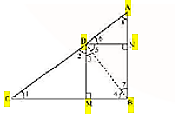Guru

# In Fig. 6.57, D is a point on hypotenuse AC of ∆ABC, such that BD ⊥AC, DM ⊥ BC and DN ⊥ AB. Prove that: (i) DM2 = DN . MC (ii) DN2 = DM . AN. Q.2

• 0

What is the best way to solve the problem of class 10th math of Triangles chapter of exercise 6.6 of question no.2 Please give me the best way to solve this problem in easy way In Fig. 6.57, D is a point on hypotenuse AC of ∆ABC, such that BD ⊥AC, DM ⊥ BC and DN ⊥ AB. Prove that: (i) DM2 = DN . MC (ii) DN2 = DM . AN.

Share

1. Let us join Point D and B.Given,

BD ⊥AC, DM ⊥ BC and DN ⊥ AB

Now from the figure we have,

DN || CB, DM || AB and ∠B = 90 °

Therefore, DMBN is a rectangle.

So, DN = MB and DM = NB

The given condition which we have to prove, is when D is the foot of the perpendicular drawn from B to AC.

∴ ∠CDB = 90° ⇒ ∠2 + ∠3 = 90° ……………………. (i)

In ∆CDM, ∠1 + ∠2 + ∠DMC = 180°

⇒ ∠1 + ∠2 = 90° …………………………………….. (ii)

In ∆DMB, ∠3 + ∠DMB + ∠4 = 180°

⇒ ∠3 + ∠4 = 90° …………………………………….. (iii)

From equation (i) and (ii), we get

∠1 = ∠3

From equation (i) and (iii), we get

∠2 = ∠4

In ∆DCM and ∆BDM,

∴ ∆DCM ∼ ∆BDM (AA similarity criterion)

BM/DM = DM/MC

DN/DM = DM/MC (BM = DN)

⇒ DM2 = DN × MC

Hence, proved.

(ii) In right triangle DBN,

∠5 + ∠7 = 90° ……………….. (iv)

In right triangle DAN,

∠6 + ∠8 = 90° ………………… (v)

D is the point in triangle, which is foot of the perpendicular drawn from B to AC.

∴ ∠ADB = 90° ⇒ ∠5 + ∠6 = 90° ………….. (vi)

From equation (iv) and (vi), we get,

∠6 = ∠7

From equation (v) and (vi), we get,

∠8 = ∠5

In ∆DNA and ∆BND,